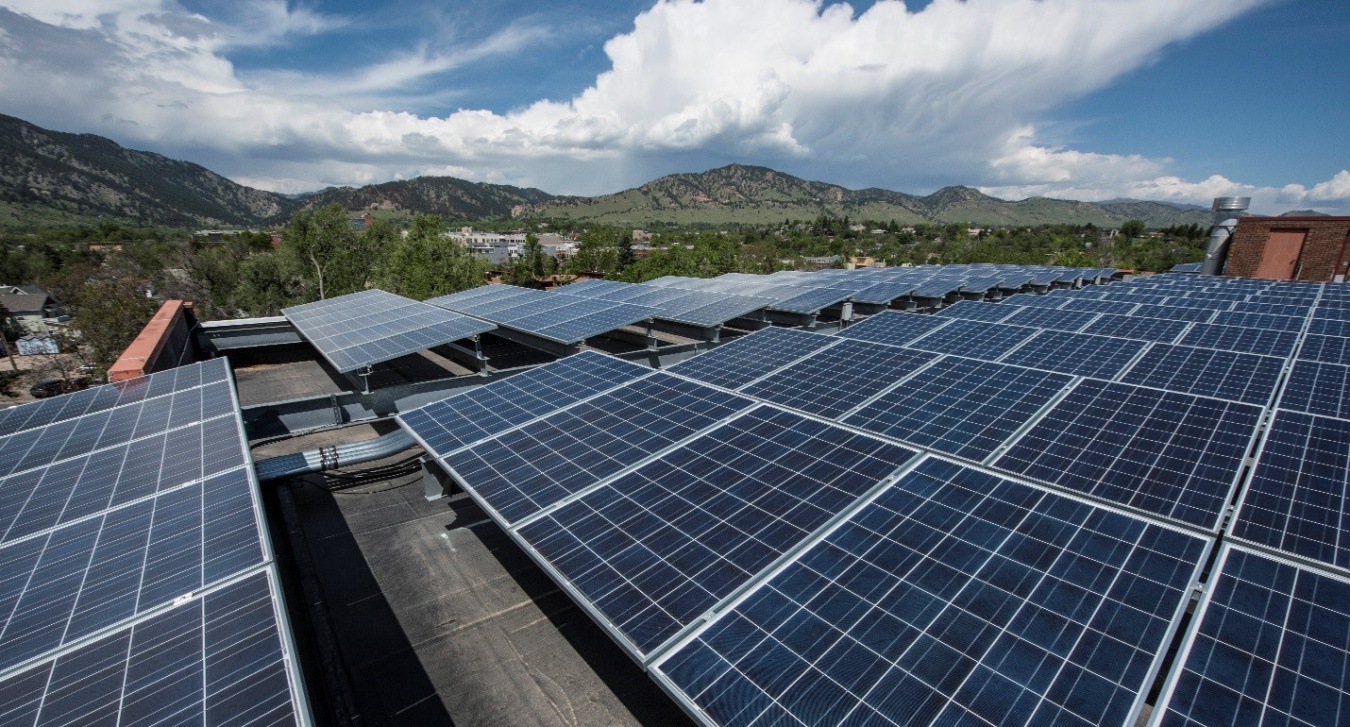What’s the difference between the installed capacity and electricity generation of energy sources?

It’s a good question and one that’s commonly misunderstood.

In the energy world, these two terms are often used to describe the growth of energy resources in the United States.

Take wind or solar, for example.According to the EIA, around 1% of U.S. electricity generation came from solar energy in 2016.

NREL

There might be an article about wind making up 8% of all energy generation capacity. Or, that solar will make up 1% of electricity generation in a specific year.

So what’s the difference? Let’s break it down for you.

### What is Capacity?

The U.S. Energy Information Administration (EIA) refers to capacity as the maximum output of electricity that a generator can produce under ideal conditions. Capacity levels are normally determined as a result of performance tests and allow utilities to project the maximum electricity load that a generator can support. Capacity is generally measured in megawatts or kilowatts.

Let’s look at an example.

According to EIA, wind turbines accounted for 8% of U.S. installed electricity generation “capacity,” as of December 2016. This means under ideal conditions, utilities would be able to supply 8% of the country’s electricity needs with wind power, but this won’t necessarily be the actual amount of electricity produced.According to EIA, wind turbines accounted for 8% of U.S. installed electricity generation capacity as of December 2016.

NREL

### What is Generation?

Electricity generation, on the other hand, refers to the amount of electricity that IS produced over a specific period of time. This is usually measured in kilowatt-hours, megawatt-hours, or terawatt-hours (1 terawatt equals 1 million megawatts). To understand the unit of megawatt-hours (MWh), consider a wind turbine with a capacity of 1.5 megawatts that is running at its maximum capacity for 2 hours. In this scenario, at the end of the second hour, the turbine would have generated 3 megawatt-hours of energy (i.e. 1.5 megawatts X 2 hours).

If the wind was not blowing strongly enough for the turbine to operate at its maximum capacity, and the same turbine was only producing 1 megawatt of power for 2 hours, the total energy generation would be 2 megawatt-hours (i.e. 1 megawatt X 2 hours). This simple thought exercise demonstrates how calculations of generation take into account the fact that not all generation sources are operating at their maximum capacity at all times, such as when the sun isn't shining or when the wind isn't blowing.